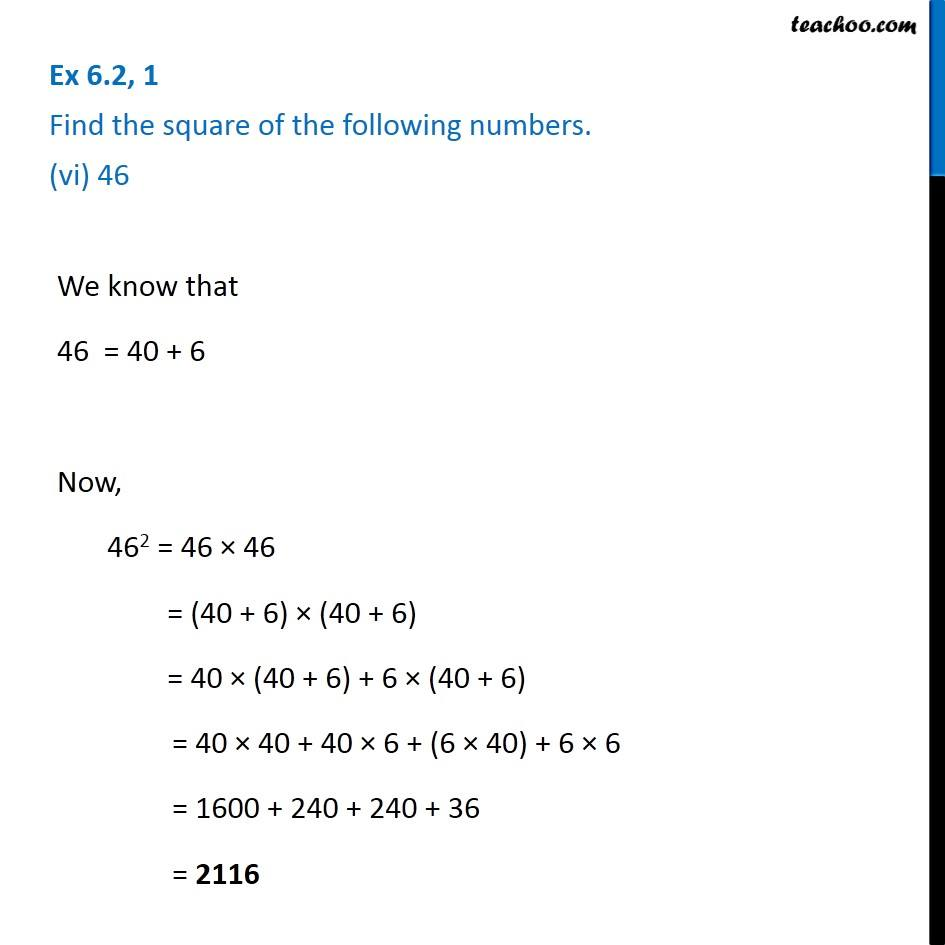Ex 6.2

Chapter 6 Class 8 Squares and Square Roots
Serial order wiseGet live Maths 1-on-1 Classs - Class 6 to 12

### Transcript

Ex 6.2, 1 Find the square of the following numbers. (vi) 46 We know that 46 = 40 + 6 Now, 462 = 46 × 46 = (40 + 6) × (40 + 6) = 40 × (40 + 6) + 6 × (40 + 6) = 40 × 40 + 40 × 6 + (6 × 40) + 6 × 6 = 1600 + 240 + 240 + 36 = 2116